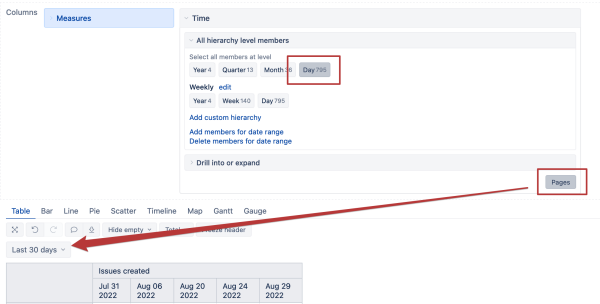# Report with calculated measures over time

I am trying to create a report that looks at the Triage SLA percentage of met for the last 50 days per Org, and provides a breakdown of how this percentage changes day to day, week to week, month to month, and quarter to quarter with the last 50 days

I have imported the data and created a calculated measure under the Triage SLA Status dimension that calculates the percentage. [Triage SLA Status].[SLA Percentage]. I am having difficulty extrapolating this percentage across time following these guidelines: Calculated members in Time dimension
I was hoping to be able to create a calculated measure that uses [Triage SLA Status].[SLA Percentage] - ( [Triage SLA Status].[SLA Percentage], ParallelPeriod([Time].[Day, 1, [Time].CurrentMember)) to create a new value that will be a dynamic reflection of percentage change from yesterday vs today, looking across the past 50 days. Is it possible to use calculated measures across the time dimension?

Hi,

The parallel period function cannot work with the aggregated members in the time dimension. If your use case compares data with one day before, you can refer to the previous member in the time dimension, which requires the standard (not aggregated) time dimension members in the report columns.

``````[Triage SLA Status].[SLA Percentage] -
( [Triage SLA Status].[SLA Percentage], [Time].CurrentHierarchyMember.PrevMember)
``````

One more comment about this formula; you should explicitly refer to a measure in the formula. The Issues created measure is assumed by default if you use the dimension members only.Kindly,
Janis, eazyBI support